Courses

# Civil Engineering (CE) Notes | EduRev

## Civil Engineering (CE): Civil Engineering (CE) Notes | EduRev

The document Civil Engineering (CE) Notes | EduRev is a part of the Civil Engineering (CE) Course Transportation Engineering.
All you need of Civil Engineering (CE) at this link: Civil Engineering (CE)

INTRODUCTION

Models are necessary to simulate the real world scenario to some extent, or in some cases, they can even provide the exact scenario. Characteristics of traffic changes with time, place, human behavior etc. The models in traffic engineering are necessary to predict the behavior of traffic in proper planning and design of the road network. The models can be microscopic and macroscopic. In the present chapter, a macroscopic model has been discussed known as cell transmission model which tries to simulate the traffic behavior.

In the classical methods to explain macroscopic behaviour of traffic, like hydrodynamic theory, differential equations need to be solved to predict traffic evolution. However in situations of sudden high density variations, like bottle-necking, the hydrodynamic model calls for a shock wave (an ad-hoc). Hence these equations are essentially piecewise continuous which are difficult to solve. Cell transmission models are developed as a discrete analogue of these differential equations in the form of difference equations which are easy to solve and also take care of high density changes.

In this lecture note the hydrodynamic model and cell transmission model and their equivalence is discussed. The cell transmission model is explained in two parts, first with only a source and a sink, and then it is extended to a network. In the first part, the concepts of basic flow advancement equations of CTM and a generalized form of CTM are presented. In addition, the phenomenon of instability is also discussed. In the second segment, the network representation and topologies are established, after which the model is discussed in terms of a linear program formulation for merging and diverging.

Single source and sink CTM model

Basic Premise

The cell transmission model simulates traffic conditions by proposing to simulate the system with a time-scan strategy where current conditions are updated with every tick of a clock. The road section under consideration is divided into homogeneous sections called cells, numbered from i = 1 to I. The lengths of the sections are set equal to the distances travelled in light traffic by a typical vehicle in one clock tick. Under light traffic condition, all the vehicles in a cell can be assumed to advance to the next with each clock tick. i.e,

ni+1(t + 1) = ni(t)                                                                 (18.1)

where, ni(t) is the number of vehicles in cell i at time t. However, equation 18.1 is not reasonable when flow exceeds the capacity. Hence a more robust set of flow advancement equations are presented in a later section.

First, two constants associated with each cell are defined, they are: (i) Ni(t) which is the maximum number of vehicles that can be present in cell i at time t, it is the product of the cell’s length and its jam density. (ii) Qi(t) : is the maximum number of vehicles that can flow into cell i when the clock advances from t to t + 1 (time interval t), it is the minimum of the capacity of cells from i − 1 and i. It is called the capacity of cell i. It represents the maximum flow that can be transferred from i − 1 to i. We allow these constants to vary with time to be able to model transient traffic incidents. Now the flow advancement equation can be written as, the cell occupancy at time t + 1 equals its occupancy at time t, plus the inflow and minus the outflow; i.e.,

ni(t + 1) = ni(t) + yi(t) − yi+1(t)                                         (18.2)

where, ni(t + 1) is the cell occupancy at time t + 1, ni(t) the cell occupancy at time t, yi(t) is the inflow at time t, yi+1(t) is the outflow at time t. The flows are related to the current conditions at time t as indicated below:

yi(t) = min [ni−1(t), Qi(t), Ni(t) − ni(t)]                             (18.3)

where, ni−1(t): is the number of vehicles in cell i − 1 at time t, Qi(t): is the capacity flow into i for time interval t, Ni(t) - ni(t): is the amount of empty space in cell i at time t.

Boundary conditions

Boundary conditions are specified by means of input and output cells. The output cell, a sink for all exiting traffic, should have infinite size (NI+1 = ∞) and a suitable, possibly time-varying, capacity. Input flows can be modeled by a cell pair. A source cell numbered 00 with an infinite number of vehicles (n00(O) = ∞) that discharges into an empty gate cell 00 of infinite size, N0(t) = ∞. The inflow capacity Q0(t) of the gate cell is set equal to the desired link input flow for time interval t + 1.

Equivalence with Hydrodynamic theory

Consider equations 18.2 & 18.3, they are discrete approximations to the hydrodynamic model with a density- flow (k-q) relationship in the shape of an isoscaled trapezoid, as in Fig.18:2. This relationship can be expressed as:

q = min [vk, qmax, v(kj − k)], for 0 ≤ k ≤ kj ,                                                 (18.4)

Flow conservation is given by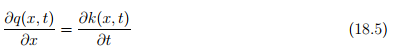To demonstrate the equivalence of the discrete and continuous approaches, the clock tick set to be equal to ∂t and choose the unit of distance such that v∂t = 1. Then the cell length is 1, v is also 1, and the following equivalences hold: x ≡ i, kj ≡ N, qmax ≡ Q, and k(x, t) ≡ ni(t) with these conventions, it can be easily seen that the equations 18.4 & 18.3 are equivalent. Equation 18.6 can be equivalently written as:

yi(t) − yi+1(t) = −ni(t) + ni+1(t + 1)                                                                       (18.6)

This represents change in flow over space equal to change in occupancy over time. Rearranging terms of equation 18.7 we can arrive at equation 18.3, which is the same as the basic flow advancement equation of the cell transmission model.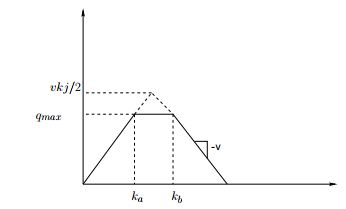Figure 18:2: Flow-density relationship for the basic cell-transmission model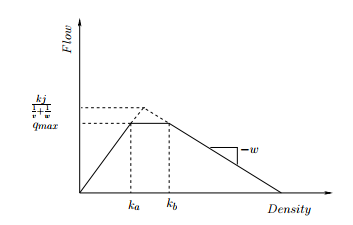Figure 18:3: Flow-density relationship for the generalized CTM

Generalized CTM Generalized CTM is an extension of the cell transmission model that would approximate the hydrodynamic model for an equation of state that allows backward waves with speed w ≤ v (see Fig. 18:3 ). This is a realistic model, since on many occasions speed of backward wave will not be same as the free flow speed.

yi(t) = min [ni−1(t), Qi(t), w/v{Ni(t) − ni(t)}]                                              (18.7)

where, α = 1 if ni−1(t) ≤ Qi(t), and α = w/v if ni−1(t) > Qi(t)

Numerical example

Consider a 1.25 km homogeneous road with speed v = 50 kmph, jam density kj = 180 veh/km and qmax = 3000 veh/hr. Initially traffic is flowing undisturbed at 80% of capacity: q = 2400 veh/hr. Then, a partial lane blockage lasting 2 min occurs on 1/3 rd of the distance from the end of the road. The blockage effectively restricts flow to 20% of the maximum. Clearly, a queue is going to build and dissipate behind the restriction. After 2 minutes, the flow in cell 3 is maximum possible flow. Predict the evolution of the traffic. Take one clock tick as 30 seconds.

Solution The main purpose of cell transmission model is to simulate the real traffic conditions for a defined stretch of road. The speed and cell length is kept constant and also the cell lengths in cell transmission model. The solution has been divided into 4 steps as follows:

Step 1: Determination of cell length and number of cells Given clock tick, t = 30sec = 1/120th of an hour. So, cell length = distance travelled by vehicle in one clock tick
= v × t = 50 × (1/120) ≈ 5/12 km. Road stretch given = 1.25 km. Therefore, no of cells = 1.25/(5/12) = 3 cells

Step 2: Determination of constants (N & Q) N = maximum number of vehicles that can be at time t in cell i, = cell length x jam density, = 180 x (5/12) = 75 vehicles, Q =
maximum number of vehicles that can flow into cell I from time t to t+1, = 3000 x (1/120) = 25 vehicles. Now, to simulate the traffic conditions for some time interval, our main aim is to find the occupancies of the 3 cells (as calculated above) with the progression of clock tick. This is easily showed by creating a table. First of all, the initial values in the tables are filled up.

Step 3: Determination of cell capacity in terms of number of vehicles for various traffic flows. For 20% of the maximum = 600 × (1/120) vehicles. For 80% of the maximum =
2400 × (1/120) vehicles.

Step 4: Initialization of the table The table has been prepared with source cell as a large capacity value and a gate is there which connects and regulates the flow of vehicles from source to cell 1 as per the capacity of the cell for a particular interval. The cell constants (Q and N) for the 3 cells are shown in the table. Note that the sink can accommodate maximum number of vehicles whichever the cell 3 generates. Q3 is the capacity in terms of number of vehicles of cell 3 . The value from H5 to H7 (i.e 5) corresponds to the 2min time interval with 4 clock ticks when the lane was blocked so the capacity reduced to 20% of the maximum (i.e. 600 × (1/120) vehicles). After the 2 min time interval is passed vehicles flows with full capacity in cell 3. So the value is 25 (i.e 3000 × (1/120) vehicles).

Step 5: Computation of Occupancies Simulation need not be started in any specific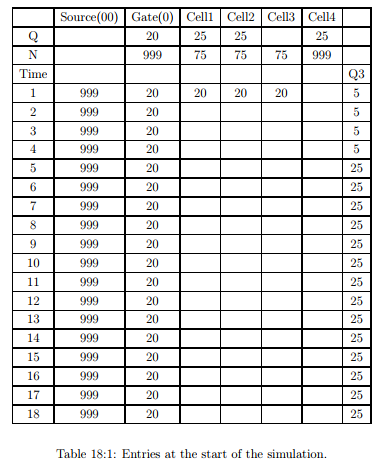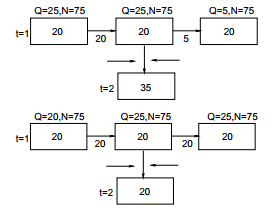order, it can be started from any cell in the row corresponding to the current clock tick. Now, consider cell circled (cell 2 at time 2) in the final table. Its entry depends on the cells marked with rectangles. By flow conservation law: Occupancy = Storage + Inflow - Outflow. Note that the Storage is the occupancy of the same cell from the preceding clock tick. Also outflow of one cell is equal to the inflow of the just succeeding cell. Here, Storage = 20. For inflow use equation 18.3 Inflow= min [20,min(25,25),(75-20)]= 20. Outflow= min [20,min(25,5),(75-20)]= 5. Occupancy= 20+20-5=35. Now, For cell 1 at time 2, Inflow= min [20,min(25,25),(75-20)]= 20, Outflow= min [20,min(25,25),(75-20)]= 20, Occupancy= 20+20-20=20. Now, For cell 3 at time 2, Inflow= min [20, min (25,5),(75-20)]= 5. Outflow= 20 (:.sink cell takes all the vehicles in previous cell) Occupancy= 20+5-20=5. Similarly, rest of the entries can be filled and the final result is shown in Table below. From the table it can be seen that the occupancy i.e. the number of vehicles on cell 1 and 2 increases and then decreases simulating the effect of lane blockage in cell 3 on cell 1 and cell 2. The lane blockage lasts 2 minutes in this problem, after that there is no congestion taken into account. So as the time passes by, the occupancy in cell 1 and cell 2 also gets reduced.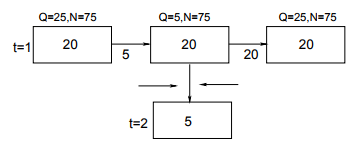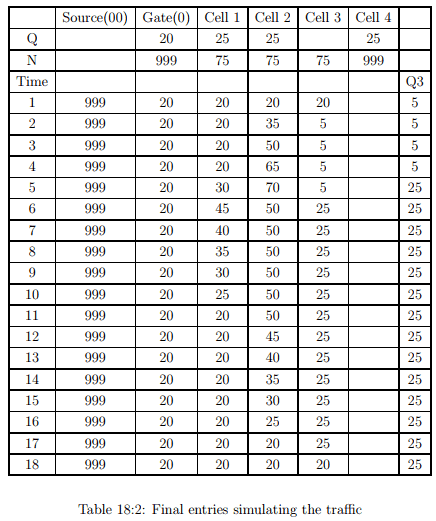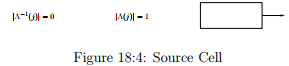Numerical example
Consider a 1.25 km homogeneous road with speed v = 50 kmph, jam density kj = 180 veh/km and qmax = 3000 veh/hr. Initially traffic is flowing undisturbed at 80% of capacity: q = 2400 VPH. Then, a partial lane blockage lasting 2 min occurs l/3 of the distance from the end of the road. The blockage effectively restricts flow to 20% of the maximum. Clearly, a queue is going to build and dissipate behind the restriction. Predict the evolution of the traffic. Take one clock tick as 6 seconds.

Solution This problem is same as the earlier problem, only change being the clock tick. This problem has been solved in Excel. The simulation is done for this smaller clock tick; the results are shown in Fig. 18:3 One can clearly observe the pattern in which the cells are getting updated. After the decrease in capacity on last one-third segment queuing is slowly building up and the backward wave can be appreciated through the first arrow. The second arrow shows the dissipation of queue and one can see that queue builds up at a faster than it dissipates. This simple illustration shows how CTM mimics the traffic conditions.

CTM: Network Traffic
General

As sequel to his first paper on CTM, Daganzo (1995) published first paper on CTM applied to network traffic. In this section application of CTM to network traffic considering merging and diverging is discussed. Some basic notations: (The notations used from here on, are adopted from Ziliaskopoulos (2000)) Γ−1 = Set of predecessor cells. Γ = Set of successor cells.

Network topologies
The notations introduced in previous section are applied to different types of cells, as shown in Figures 18:4, 18:5 & 18:6. Some valid and invalid representations in a network are shown in Fig 18:10 & Fig 18:9.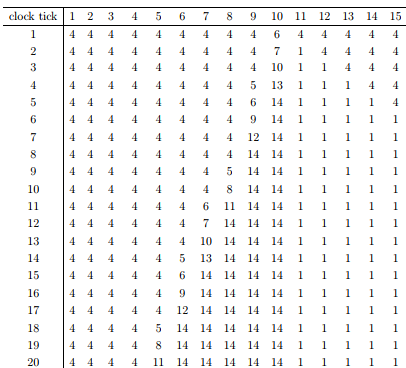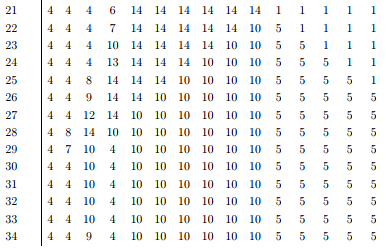Table 18:3: Illustration of traffic simulation in CTM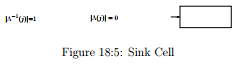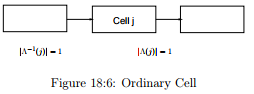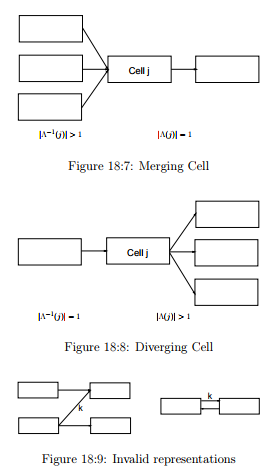yk(t) = min(nBk(t), min[QBk(t), QEk(t)], δEk[NEk(t) − nEk(t)]) (18.9) where, δ = w/v. yk(t) is the inflow to cell Ek in the time interval (t,t + 1). Defining the maximum flows that can be sent and received by the cell i in the interval between t to t + 1 as SI (t) = min(QI, nI), and RI (t) = min(QI , δI , [NI − nI ]). Therefore, yk(t) can be written in a more compact form as: yk(t) = min(SBk, REk). This means that the flow on link k should be the maximum that can be sent by its upstream cell unless prevented to do so by its end cell. If blocked in this manner, the flow is the maximum allowed by the end cell. From equations one can see that a simplification is done by splitting yk(t) in to SBk and REk terms. ’S’ represents sending capacity and ’R’ represents receiving capacity. During time periods when SBk< REkthe flow on link k is dictated by upstream traffic conditions-as would be predicted from the forward moving characteristics of the Hydrodynamic model. Conversely, when SBk > REk, flow is dictated by downstream conditions and backward moving characteristics.

Merging and Diverging

Consider two cells merging, here we have a beginning cell and its complimentary merging into ending cell, the constraints on the flow that can be sent and received are given by equation 18.10 and equation 18.10.

yk(t) ≤ SBk; yck(t) ≤ Sckyk(t) + yck(t) ≤ REk                                                        (18.10)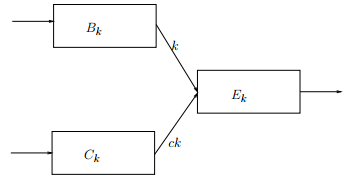where, SI (t) = (QI , nI ), and RI (t) = (QI , δI, [NI − nI ]). A number of combinations of yk(t) + yck(t) are possible satisfying the above said constraints. Similarly for diverging a number of possible outflows to different links is possible satisfying corresponding constraints, hence this calls for an optimization problem. Ziliaskopoulos (2000), has given this LP formulations for both merging and diverging, this has been discussed later

CTM Software - NETCELL

• NETCELL is a freeway network simulation program based on the cell transmission model developed by Cay ford, Lin and Daganzo.

• It consists of two components,

– NETCELL

– NETVIEW(a graphical post-processor)

• NETCELL is a macroscopic simulation program in which vehicle quantities are treated as continuous variables. Vehicles are advanced in a way consistent with the hydrodynamic theory of traffic flow.

The document Civil Engineering (CE) Notes | EduRev is a part of the Civil Engineering (CE) Course Transportation Engineering.
All you need of Civil Engineering (CE) at this link: Civil Engineering (CE)Use Code STAYHOME200 and get INR 200 additional OFF Use Coupon Code
All Tests, Videos & Notes of Civil Engineering (CE): Civil Engineering (CE)

### Top Courses for Civil Engineering (CE)## Transportation Engineering

23 videos|66 docs|39 tests

### Top Courses for Civil Engineering (CE)Track your progress, build streaks, highlight & save important lessons and more!

,

,

,

,

,

,

,

,

,

,

,

,

,

,

,

,

,

,

,

,

,

;